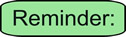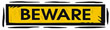Which Method Should Be Used to Solve a Quadratic Equation? MathBitsNotebook.com Terms of Use   Contact Person: Donna RobertsQuadratic equations are of the form ax2 + bx + c = 0 where a, b and c are real numbers, a ≠ 0. Quadratic equations have two solutions, but it is possible that one solution may repeat.

 Some questions will indicate which method of solution to use when solving a quadratic equation, but other questions will leave the choice of method to you. So, which method is the "best" method to use? Some students believe that since the "quadratic formula" can be used on ALL quadratic equations, it is the "best" (most appropriate) method for ALL problems. Even though the quadratic formula is a fabulous formula, it can be "overkill" (burdensome) for certain problems. Why do extra work, if a simpler method could be faster (more efficient).Ways to Solve Quadratic Equations and When to Use Them:Factoring Method:Square Root Method: Use when: 1. you are told to solve by factoring.      Such as:  "Solve by factoring". 2. the quadratic is easily factorable.      Such as:   x2 - 4x - 12 = 0 3. the quadratic is already factored.      Such as:   (x + 5)(x - 8) = 0 4. the constant term, c, is missing.      Such as:  3x2 - x = 0 Use when: 1. you are told to solve by square root method.      Such as:  "Solve by square root method". 2. x2 is set equal to a numeric value.      Such as:  x2 = 9 or x2 = 12 3. the middle term, bx, is missing.      Such as:  3x2 - 15 = 0 4. you have the difference of two squares.      Such as:  x2 - 81 = 0Completing the Square Method:Quadratic Formula Method: Use when: 1. you are told to solve by completing the square. Such as:  "Solve by completing the square". 2. you are told to put the quadratic into vertex form, a(x - h)2 + k = 0, before solving. Use when: 1. you are told to use the quadratic formula.      Such as:  "Solve by the quadratic formula". 2. factoring looks difficult, or you are having trouble finding the correct factors.      Such as:   10x2 - 3x - 4 = 0 3. the quadratic is not factorable.      Such as:  x2 - 6x + 2 = 0 4. the question asks for the answers to ax2 + bx + c = 0 to be rounded.      Such as:   2x2 + 18x + 4 = 0 5. the question asks for the answers to be written in a+bi form.       Such as:  x2 - 6x + 2 = 0Graphing Quadratic Equations: If you are graphing to FIND the zeros of the equation, then you are using a graphing utility (calculator). Any other method would have you finding the zeros BEFORE you draw the graph. (Yes, the vertex method would create a graph without knowing the roots, but most likely you would still not know the roots.) Use when: 1. you have a graphing utility (calculator) with the capability of finding the decimal values (approximations) of non-integer (unfriendly) roots (zeros). If the roots are not obviously appearing as integer values on the x-axis of the graph, then use the graphing calculator's capabilities to determine the decimal values of the roots (usually a "Calculate ZERO" option on the calculator).Remember, if your graph does not cross the x-axis, you will be dealing with complex roots and you must use a different method to find those roots. Such a method will be discussed in Algebra 2.